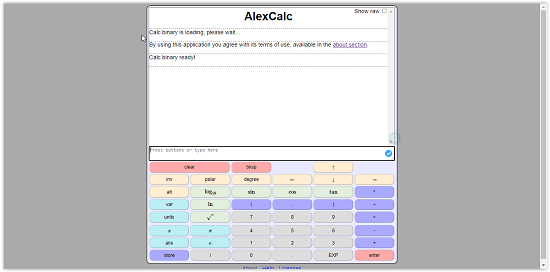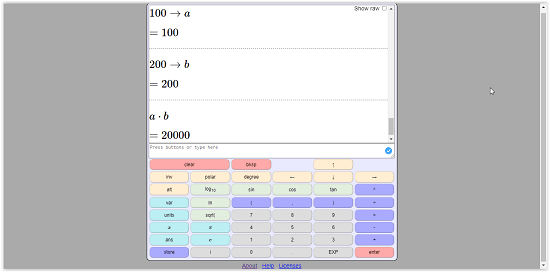Editor Ratings:
User Ratings:
[Total: 0 Average: 0]

AlexCalc is a free online scientific calculator. The scientific calculator also has a LaTeX equation display along with all the other features of a usual scientific calculator. They also have the tool ready for Android and IOS.

Writing documents having multiple various mathematical functions is a tough task. You need to watch over a lot of things. To create this type of document in a word processor is a very rigid task. And even using the scientific calculator for multiple calculations is tough. You need to write the precise formulae and functions every time. So today I have written a post about the solution to these problems, which is AlexCalc. It uses LaTeX, which is a primary choice for writing technical documents. LaTeX lets you write mathematical functions easily. Let’s see how is this helpful.

## How to use AlexCalc

Visit the website of AlexCalc. Clicking on this given link will directly open the calculator for you. There are virtual keys on the screen for functions. But you can also use your keyboard. The home screen of the website looks like this:Let’s break the process into steps.

Step-1: Open the website and start writing

The calculator has a writing bar just above the virtual keys. Here, you type the mathematical equations. But unlike other calculators, LaTeX makes writing these formulae and functions easy. Now you don’t have to write the usual symbols. There are many other features given below:

• Storing values to variables: The calculator gives you an option to store the value of a variable. Just type a value, then click on the store button (bottom left corner). Then type a variable and hit enter. The value is stored in the variable. Now you can just call the variable any time.
• Units and conversion: The output given by the website is in SI units. But you can convert the units using the “to” operator. For example, you can convert kilogram to gram by simply typing 1 kg to g. And the website will convert 1 kg to g and show you 1000g as result. Other similar conversions are also available.

Step-2: Write and solve your mathematical problems

Solving math problems was never easier before. You just need to write how you want to solve the problem, and it will be solved. No unnecessary use of typical mathematical functions and symbols is required. You can use an easier alphabetical method to do it. I have added a screenshot below as an example:In the above image, you can see:

• 100 is stored in variable a
• 200 is stored in variable b
• A multiplication function between a and b

### Conclusion:

AlexCalc is a very useful tool. And not just for the technical people. But for normal users as well. I liked the tool because it makes the work a lot easier. Although it is quite scientific, hence a little tough to understand initially. Therefore, it might sound not useful for many users. And boring too. But the good thing is that it is useful. And it is free to use.